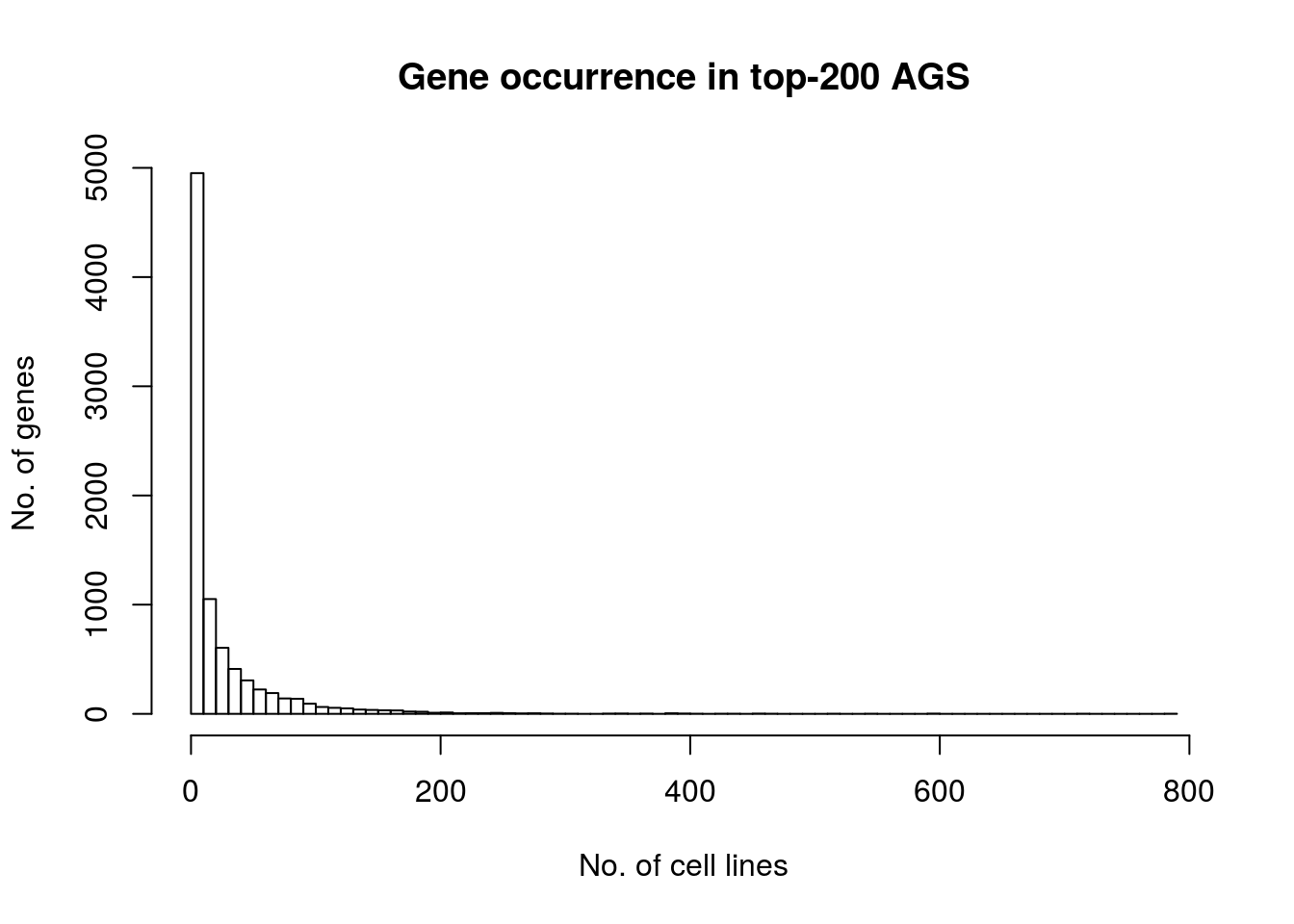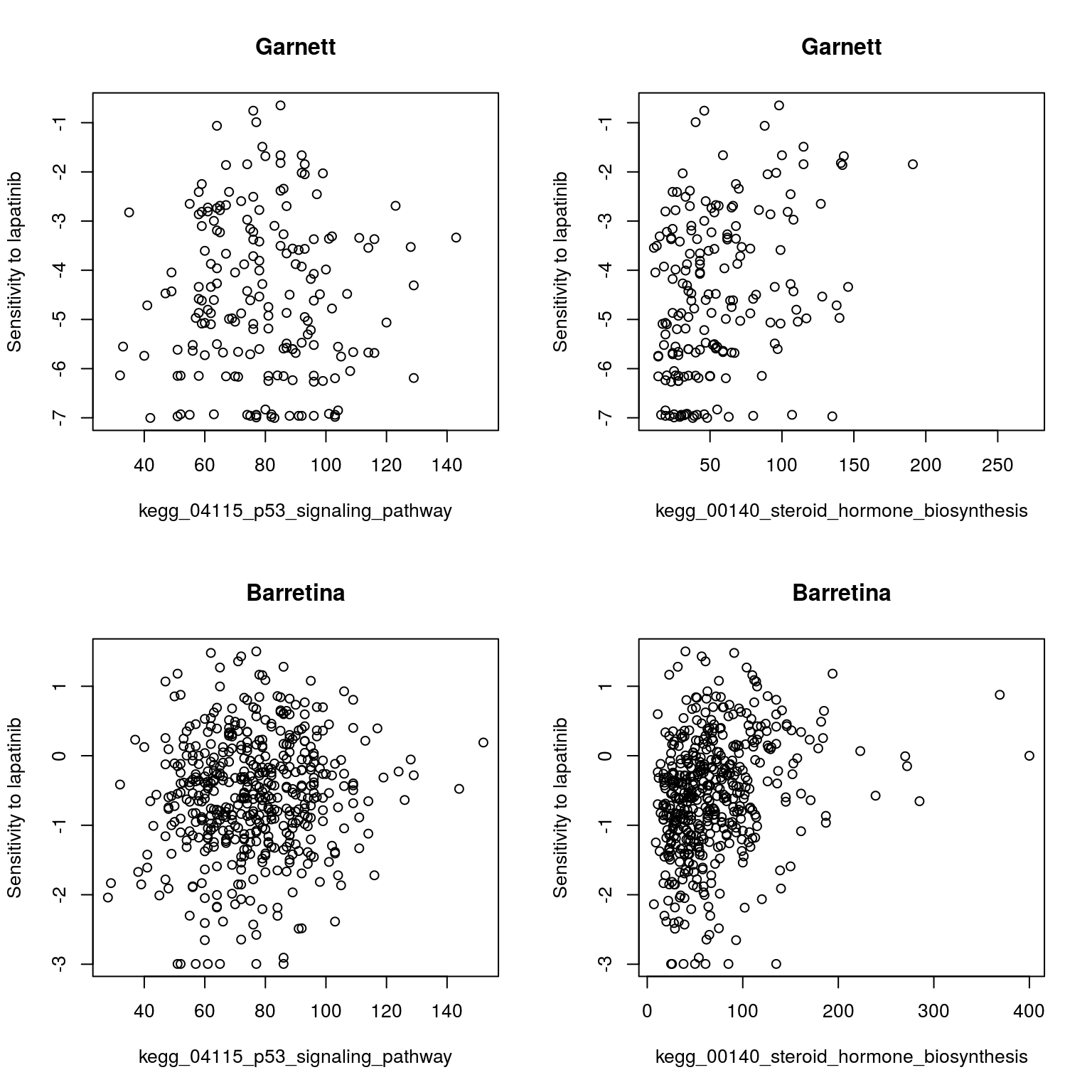How to use stand-alone R software for NEA

The same procedures that run at the web site can be more systematically implemented using the package NEArender. It contains basic functions for network and gene set enrichment analyses, creating, re-formatting, and saving gene set lists, as well as benchmarking the networks.

Installation of NEArender package
NEArender can be installed from R CRAN directly within R environment. The only recommendation is to install first the required package ROCR (it might be sensitive to some other dependencies).

``````install.packages("ROCR",repos="http://cran.rstudio.com/")
install.packages("NEArender",repos="http://cran.rstudio.com/")
library("ROCR")
library("NEArender")``````

Create Altered Gene Sets (AGS) input file
We first could create a set of AGS that distinguish each cell line sample in the collection from the others.

``````download.file("http://research.scilifelab.se/andrej_alexeyenko/downloads/evinet/Data.BRCA_and_CTD.June2015.RData.gz","Data.BRCA_and_CTD.June2015.RData.gz")
Data <- NULL;
Data\$CTD <- d1.ctd;
m0 <- Data\$CTD\$GE\$Affymetrix1;
rm(d1.ctd);
rm(d1.brca);
AGS <- samples2ags(m0, Ntop=200, method="top", Lowercase = 1);
save_gs_list(AGS, File="CTD.Affy1.top200.txt");
hist(table(unlist(AGS)), breaks=100, main="Gene occurrence in top-200 AGS", xlab="No. of cell lines", ylab="No. of genes");``````Importing Functional Gene Sets (FGS) and Networks
Plotting the gene frequency distribution demonstrates that the most of the genes are quite unique (below). Next, we run the network enrichment analysis, analyzing enrichment of each of these cell-line specific AGSs against each of the 330 pathways in the FGS collection

``````download.file("http://research.scilifelab.se/andrej_alexeyenko/downloads/evinet/Related_and_CAN_MET_SIG_GO2","Related_and_CAN_MET_SIG_GO2")
FGS <- import.gs ("Related_and_CAN_MET_SIG_GO2", Lowercase=1, col.gene= 2, col.set= 3, gs.type= 'f');
NET <- import.net("merged6_and_wir1_HC2");``````
``##  "Network of 971577 edges between 19027 nodes..."``
``Data\$CTD\$NEA\$top.200.affymetrix1 <- nea.render(AGS = AGS, FGS = FGS, NET = NET, echo=1, Parallelize=1);``
``````##  "Preparing input datasets:"
##  "Network: 19027 genes/proteins."
##  "FGS: 6299 genes in 328 groups."
##  "AGS: 8552 genes in 1034 groups..."
##  "Calculating N links expected by chance..."
##     user   system  elapsed
## 1541.542    0.000 1541.201
##  "Calculating statistics..."
##  "Done."``````

top

Run NEA
We now are in the position to identify pathway -level correlates of drug sensitivity, such as these two pathways associated with resistance to lapatinib . Furthermore, this correlation can be traced between two independent drug screens (Barretina et al., 2012 and Garnett et al., 2012) . While using original gene expression values, such correlation would be much weaker

``````commonIdx <- function(m1, m2, Dir) {
if (is.null(Dir) | !grepl("col|vec|row", Dir, ignore.case = T, perl=T)) {
stop("The 3rd argument should be one of: vector (vec), column (col), rows (row)...");
}
if (grepl("row", Dir, ignore.case = T, perl=T)) {
vec1 <- rownames(m1);
vec2 <- rownames(m2);
}
if (grepl("col", Dir, ignore.case = T, perl=T)) {
vec1 <- colnames(m1);
vec2 <- colnames(m2);
}
if (grepl("vec", Dir, ignore.case = T, perl=T)) {
vec1 <- as.vector(m1);
vec2 <- as.vector(m2);
}

t1 <-   table(c(vec1, vec2));
common <- names(t1)[which(t1 > 1)];
common <- common[which(common %in% vec1)];
common <- common[which(common %in% vec2)];
return(common);
}``````
``````d1 <- "lapatinib";
sc1 <- "Garnett";
sc2 <- "Barretina";
drug1 <- Data\$CTD\$CLIN\$DRUGSCREEN[[sc1]];
drug2 <- Data\$CTD\$CLIN\$DRUGSCREEN[[sc2]];
expr <- Data\$CTD\$NEA\$top.200.affymetrix1\$n.actual;
usedSamples1 <- commonIdx (drug1, expr, Dir="col");
usedSamples2 <- commonIdx(drug2, expr, Dir="col");
pw1 <- "kegg_04115_p53_signaling_pathway";
pw2 <- "kegg_00140_steroid_hormone_biosynthesis";
par(mfrow=c(2,2))
plot(expr [pw1,usedSamples1], drug1[d1,usedSamples1], xlab=pw1,
ylab=paste("Sensitivity to ", d1, sep=""), main=sc1);
plot(expr[pw2,usedSamples1], drug1[d1,usedSamples1], xlab=pw2,
ylab=paste("Sensitivity to ", d1, sep=""), main=sc1);
plot(expr[pw1,usedSamples2], drug2[d1,usedSamples2], xlab=pw1,
ylab=paste("Sensitivity to ", d1, sep=""), main=sc2);
plot(expr[pw2,usedSamples2], drug2[d1,usedSamples2], xlab=pw2,
ylab=paste("Sensitivity to ", d1, sep=""), main=sc2);``````The two pathways seems to have discovered a global trend: correlation coefficients from the two drug screens correlate with each other, too.

``````plot(cor(drug1[d1,usedSamples1], t(expr[,usedSamples1]),
use="pairwise.complete.obs", method="spearman"),
cor(drug2[d1,usedSamples2], t(expr[,usedSamples2]),
use="pairwise.complete.obs", method="spearman"),
main = NA, ylab="Pathway-drug correlation in Barretina",
xlab="Pathway-drug correlation in Garnett",
col = ifelse(rownames(expr) %in% c(pw1, pw2), "red", "grey3"))``````